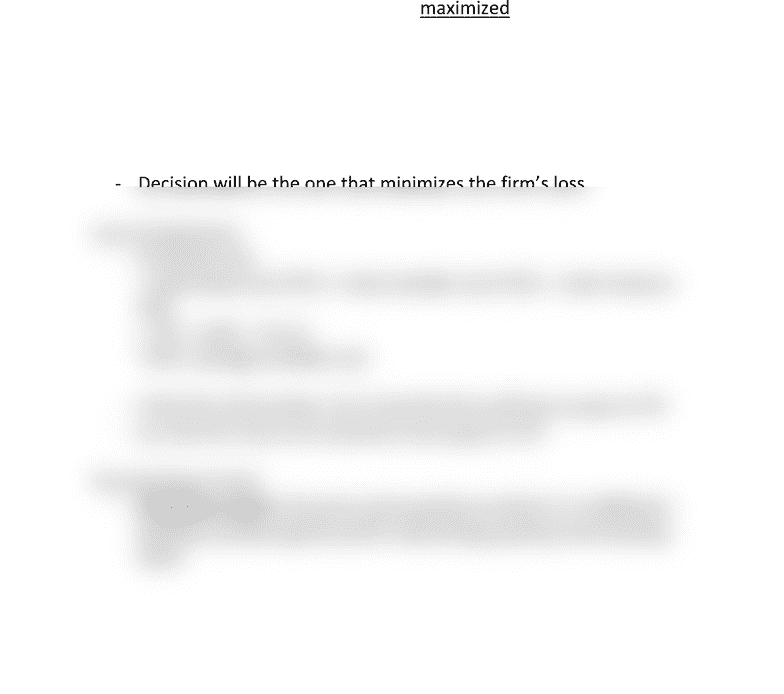Class Notes (1,100,000)
CA (650,000)
UW (20,000)
ECON (1,000)
ECON101 (500)
Lecture 9

# ECON101 Lecture Notes - Lecture 9: Average Variable Cost, Marginal Revenue, Marginal Cost

Department
Economics
Course Code
ECON101
Professor
Corey Van De Waal
Lecture
9

This preview shows half of the first page. to view the full 2 pages of the document.Marginal Analysis and Supply Decision
- Because marginal revenue is constant (horizontal) and marginal
cost eventually increases as output increases, profit is maximized
by producing the output at which
marginal revenue (MR) = marginal cost (MC)
- If MR > MC, economic profit increases if output increases
- If MR < MC, economic profit decreases if output increases
- If MR = MC, economic profit decreases if output changes in either
direction so economic profit is maximized
Temporary Shutdown Decision
- Economic loss a firm should decide whether to exit or stay in
the market
- If decide to stay must decide whether to produce something or
to shut down temporarily
- Decision will be the one that minimizes the firm’s loss
Loss Comparisons
- Economic loss
= total fixed cost (TFC) + total variable cost (TVC) total revenue
(TR)
= TFC + (AVC - P) x Q
*AVC: average variable cost
- If the firm shuts down, Q is 0 and the firm still has to pay its TFC
- So, the firm incurs an economic loss equal to TFC
The Shutdown Point
- Shutdown point: the price and quantity at which it is indifferent
between producing the profit-maximizing quantity and shutting
down